Anúncio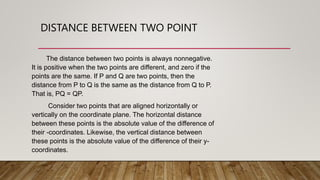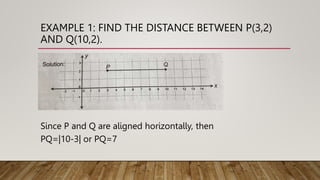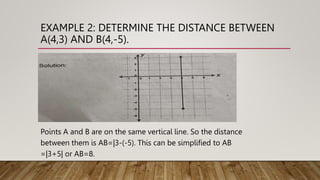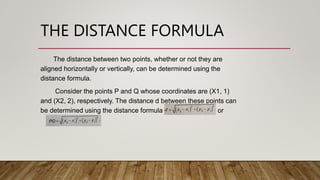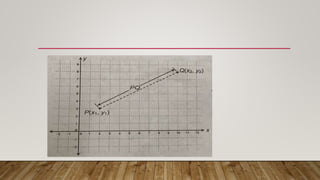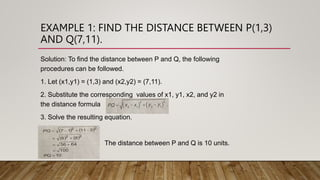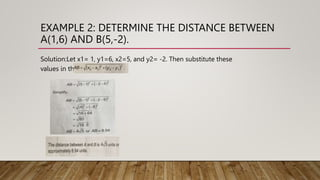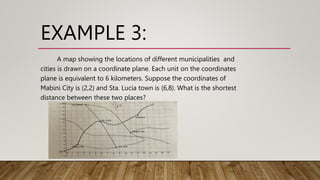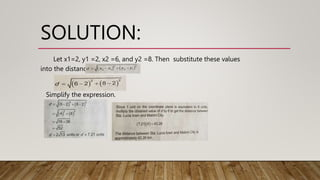1 de 11
Anúncio

### math presentation copy.pptx

1. DISTANCE BETWEEN TWO POINTS PRECIOUS FRAULEIN RODA CASSANDRA TAGULALAP SHAINA MAE FADERON
2. DISTANCE BETWEEN TWO POINT The distance between two points is always nonnegative. It is positive when the two points are different, and zero if the points are the same. If P and Q are two points, then the distance from P to Q is the same as the distance from Q to P. That is, PQ = QP. Consider two points that are aligned horizontally or vertically on the coordinate plane. The horizontal distance between these points is the absolute value of the difference of their -coordinates. Likewise, the vertical distance between these points is the absolute value of the difference of their y- coordinates.
3. EXAMPLE 1: FIND THE DISTANCE BETWEEN P(3,2) AND Q(10,2). Since P and Q are aligned horizontally, then PQ=|10-3| or PQ=7
4. EXAMPLE 2: DETERMINE THE DISTANCE BETWEEN A(4,3) AND B(4,-5). Points A and B are on the same vertical line. So the distance between them is AB=|3-(-5). This can be simplified to AB =|3+5| or AB=8.
5. THE DISTANCE FORMULA The distance between two points, whether or not they are aligned horizontally or vertically, can be determined using the distance formula. Consider the points P and Q whose coordinates are (X1, 1) and (X2, 2), respectively. The distance d between these points can be determined using the distance formula or
6. EXAMPLE 1: FIND THE DISTANCE BETWEEN P(1,3) AND Q(7,11). Solution: To find the distance between P and Q, the following procedures can be followed. 1. Let (x1,y1) = (1,3) and (x2,y2) = (7,11). 2. Substitute the corresponding values of x1, y1, x2, and y2 in the distance formula 3. Solve the resulting equation. . Add a. The distance between P and Q is 10 units.
7. EXAMPLE 2: DETERMINE THE DISTANCE BETWEEN A(1,6) AND B(5,-2). Solution:Let x1= 1, y1=6, x2=5, and y2= -2. Then substitute these values in the formula
8. EXAMPLE 3: A map showing the locations of different municipalities and cities is drawn on a coordinate plane. Each unit on the coordinates plane is equivalent to 6 kilometers. Suppose the coordinates of Mabini City is (2,2) and Sta. Lucia town is (6,8). What is the shortest distance between these two places?
9. SOLUTION: Let x1=2, y1 =2, x2 =6, and y2 =8. Then substitute these values into the distance formula Simplify the expression.
Anúncio